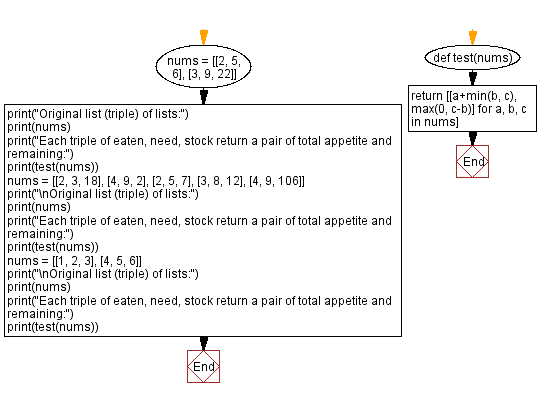﻿ Python: Each triple of eaten, need, stock return a pair of total appetite and remaining - w3resource# Python: Each triple of eaten, need, stock return a pair of total appetite and remaining

## Python Programming Puzzles: Exercise-90 with Solution

For each triple of eaten, need, stock write a Python program to get a pair of total appetite and remaining.

```Input:
[[2, 5, 6], [3, 9, 22]]
Output:
[[7, 1], [12, 13]]

Input:
[[2, 3, 18], [4, 9, 2], [2, 5, 7], [3, 8, 12], [4, 9, 106]]
Output:
[[5, 15], [6, 0], [7, 2], [11, 4], [13, 97]]

Input:
[[1, 2, 3], [4, 5, 6]]
Output:
[[3, 1], [9, 1]]
```

Sample Solution:

Python Code:

`````` #License: https://bit.ly/3oLErEI

def test(nums):
return [[a+min(b, c), max(0, c-b)] for a, b, c in nums]

nums = [[2, 5, 6], [3, 9, 22]]
print("Original list (triple) of lists:")
print(nums)
print("Each triple of eaten, need, stock return a pair of total appetite and remaining:")
print(test(nums))
nums = [[2, 3, 18], [4, 9, 2], [2, 5, 7], [3, 8, 12], [4, 9, 106]]
print("\nOriginal list (triple) of lists:")
print(nums)
print("Each triple of eaten, need, stock return a pair of total appetite and remaining:")
print(test(nums))
nums = [[1, 2, 3], [4, 5, 6]]
print("\nOriginal list (triple) of lists:")
print(nums)
print("Each triple of eaten, need, stock return a pair of total appetite and remaining:")
print(test(nums))
``````

Sample Output:

```Original list (triple) of lists:
[[2, 5, 6], [3, 9, 22]]
Each triple of eaten, need, stock return a pair of total appetite and remaining:
[[7, 1], [12, 13]]

Original list (triple) of lists:
[[2, 3, 18], [4, 9, 2], [2, 5, 7], [3, 8, 12], [4, 9, 106]]
Each triple of eaten, need, stock return a pair of total appetite and remaining:
[[5, 15], [6, 0], [7, 2], [11, 4], [13, 97]]

Original list (triple) of lists:
[[1, 2, 3], [4, 5, 6]]
Each triple of eaten, need, stock return a pair of total appetite and remaining:
[[3, 1], [9, 1]]
```

Flowchart:## Visualize Python code execution:

The following tool visualize what the computer is doing step-by-step as it executes the said program:

Python Code Editor :

Have another way to solve this solution? Contribute your code (and comments) through Disqus.

What is the difficulty level of this exercise?

Test your Programming skills with w3resource's quiz.

﻿

## Python: Tips of the Day

Clamps num within the inclusive range specified by the boundary values x and y:

Example:

```def tips_clamp_num(num,x,y):
return max(min(num, max(x, y)), min(x, y))
print(tips_clamp_num(2, 4, 6))
print(tips_clamp_num(1, -1, -6))
```

Output:

```4
-1
```